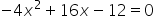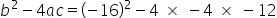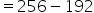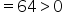Mathematics
Easy

Question

# Find the number of solutions obtained to the quadratic equation using the discriminant.

## 0    1    2    Either 0 or 1Hint:

## The correct answer is: 2

### Step 1 of 1:We have given an equationThe discriminant is the part of the quadratic formula found within the square root.

### Related Questions to study#### With Turito Foundation.#### Get an Expert Advice From Turito.